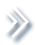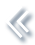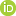####ArchivesReview Articles

## Variable selection in finite mixture of median regression models using skew-normal distribution

Xin Zeng ,

Faculty of Science, Kunming University of Science and Technology, Kunming, People's Republic of China;b School of Economics, Xiamen University, Xiamen, People's Republic of ChinaYuanyuan Ju ,

Faculty of Science, Kunming University of Science and Technology, Kunming, People's Republic of ChinaLiucang Wu

Faculty of Science, Kunming University of Science and Technology, Kunming, People's Republic of Chinawuliucang@163.com

Pages | Received 18 Apr. 2021, Accepted 25 Jul. 2022, Published online: 06 Aug. 2022,
• Abstract
• References
• Citations

A regression model with skew-normal errors provides a useful extension for traditional normal regression models when the data involve asymmetric outcomes. Moreover, data that arise from a heterogeneous population can be efficiently analysed by a finite mixture of regression models. These observations motivate us to propose a novel finite mixture of median regression model based on a mixture of the skew-normal distributions to explore asymmetrical data from several subpopulations. With the appropriate choice of the tuning parameters, we establish the theoretical properties of the proposed procedure, including consistency for variable selection method and the oracle property in estimation. A productive nonparametric clustering method is applied to select the number of components, and an efficient EM algorithm for numerical computations is developed. Simulation studies and a real data set are used to illustrate the performance of the proposed methodologies.

• Akaike, H. (1973). Information theory and an extension of the maximum likelihood principle. International Symposium on Information Theory1, 610–624. https://doi.org/10.1007/978-1-4612-1694-0_15
• Atienza, N., Garcia-Heras, J., & Muñoz-Pichardo, J. (2006). A new condition for identifiability of finite mixture distributions. Metrika63(2), 215–221. https://doi.org/10.1007/s00184-005-0013-z
• Azzalini, A. (1985). A class of distributions which includes the normal ones. Scandinavian Journal of Statistics12(2), 171–178. http://www.jstor.org/stable/4615982
• Azzalini, A., & Capitanio, A. (2013). The skew-normal and related families. Cambridge University Press.
• Chen, J. (2017). Consistency of the MLE under mixture models. Statistical Science32(1), 47–63. https://doi.org/10.1214/16-sts578
• Chen, J., Li, P., & Liu, G. (2020). Homogeneity testing under finite location-scale mixtures. Canadian Journal of Statistics48(4), 670–684. https://doi.org/10.1002/cjs.11557
• Chen, J., & Tan, X. (2009). Inference for multivariate normal mixtures. Journal of Multivariate Analysis100(7), 1367–1383. https://doi.org/10.1016/j.jmva.2008.12.005
• Cook, R.-D., & Weisberg, S. (1994). An introduction to regression graphics. John Wiley and Sons.
• Fan, J., & Li, R. (2001). Variable selection via nonconcave penalized likelihood and its oracle properties. Journal of the American Statistical Association96(456), 1348–1360. https://doi.org/10.1198/016214501753382273
• Goldfeld, S., & Quandt, R. (1973). A Markov model for switching regressions. Journal of Econometrics1(1), 3–15. https://doi.org/10.1016/0304-4076(73)90002-X
• He, M., & Chen, J. (2022a). Consistency of the MLE under a two-parameter gamma mixture model with a structural shape parameter. Metrika. https://doi.org/10.1007/s00184-021-00856-9
• He, M., & Chen, J. (2022b). Strong consistency of the MLE under two-parameter gamma mixture models with a structural scale parameter. Advances in Data Analysis and Classification16(1), 125–154. https://doi.org/10.1007/s11634-021-00472-5
• Hu, D., Gu, Y., & Zhao, W. (2019). Bayesian variable selection for median regression. Chinese Journal of Applied Probability and Statistics35(6), 594–610.
• Karlis, D., & Xekalaki, E. (2003). Choosing initial values for the EM algorithm for finite mixtures. Computational Statistics & Data Analysis41(3–4), 577–590. https://doi.org/10.1016/S0167-9473(02)00177-9
• Khalili, A., & Chen, J. (2007). Variable selection in finite mixture of regression models. Journal of the American Statistical Association102(479), 1025–1038. https://doi.org/10.1198/016214507000000590
• Kottas, A., & Gelfand, A. (2001). Bayesian semiparametric median regression modeling. Journal of the American Statistical Association96(456), 1458–1468. https://doi.org/10.1198/016214501753382363
• Li, H., Wu, L., & Ma, T. (2017). Variable selection in joint location, scale and skewness models of the skew-normal distribution. Journal of Systems Science and Complexity30(3), 694–709. https://doi.org/10.1007/S11424-016-5193-2
• Li, H., Wu, L., & Yi, J. (2016). A skew-normal mixture of joint location, scale and skewness models. Applied Mathematics-A Journal of Chinese Universities31(3), 283–295. https://doi.org/10.1007/S11766-016-3367-2
• Li, J., Ray, S., & Lindsay, B.-G. (2007). A nonparametric statistical approach to clustering via mode identification. Journal of Machine Learning Research8(8), 1687–1723.
• Lin, T.-I., Lee, J., & Yen, S. (2007). Finite mixture modelling using the skew normal distribution. Statistica Sinica17(3), 909–927. http://www.jstor.org/stable/24307705
• Liu, M., & Lin, T.-I. (2014). A skew-normal mixture regression model. Educational and Psychological Measurement74(1), 139–162. https://doi.org/10.1177/0013164413498603
• McLachlan, G., & Peel, D. (2004). Finite mixture models. John Wiley and Sons.
• Otiniano, C. E. G., Rathie, P. N., & Ozelim, L. C. S. M. (2015). On the identifiability of finite mixture of skew-normal and skew-t distributions. Statistics & Probability Letters106, 103–108. https://doi.org/10.1016/j.spl.2015.07.015
• Richardson, S., & Green, P. (1997). On bayesian analysis of mixtures with an unknown number of components (with discussion). Journal of the Royal Statistical Society: Series B (Statistical Methodology)59(4), 731–792. https://doi.org/10.1111/1467-9868.00095
• Schwarz, G. (1978). Estimating the dimension of a model. The Annals of Statistics6(2), 461–464. https://doi.org/10.1214/AOS/1176344136
• Tang, A., & Tang, N. (2015). Semiparametric Bayesian inference on skew-normal joint modeling of multivariate longitudinal and survival data. Statistics in Medicine34(5), 824–843. https://doi.org/10.1002/SIM.6373
• Tibshirani, R. (1996). Regression shrinkage and selection via the lasso. Journal of the Royal Statistical Society. Series B. Statistical Methodology58(1), 267–288. https://doi.org/10.1111/J.2517-6161.1996.TB02080.X
• Titterington, D., Smith, A., & Makov, U. (1985). Statistical analysis of finite mixture distributions. John Wiley and Sons
• Wang, H., Li, R., & Tsai, C. (2007). Tuning parameter selectors for the smoothly clipped absolute deviation method. Biometrika94(3), 553–568. https://doi.org/10.1093/BIOMET/ASM053
• Wang, P., Puterman, M., Cockburn, I., & Le, N. (1996). Mixed Poisson regression models with covariate dependent rates. Biometrics52(2), 381–400. https://doi.org/10.2307/2532881
• Wu, L. (2014). Variable selection in joint location and scale models of the skew-t-normal distribution. Communications in Statistics. Simulation and Computation43(3), 615–630. https://doi.org/10.1080/03610918.2012.712182
• Wu, L., Li, S., & Tao, Y. (2020). Estimation and variable selection for mixture of joint mean and variance models. Communications in Statistics-Theory and Methods50(24), 6081–6098. https://doi.org/10.1080/03610926.2020.1738493
• Wu, L., Zhang, Z., & Xu, D. (2013). Variable selection in joint location and scale models of the skew-normal distribution. Journal of Statistical Computation and Simulation83(7), 1266–1278. https://doi.org/10.1080/00949655.2012.657198
• Yao, W., & Li, L. (2014). A new regression model: Modal linear regression. Scandinavian Journal of Statistics41(3), 656–671. https://doi.org/10.1111/SJOS.12054
• Yin, J., Wu, L., & Dai, L. (2020). Variable selection in finite mixture of regression models using the skew-normal distribution. Journal of Applied Statistics47(16), 2941–2960. https://doi.org/10.1080/02664763.2019.1709051
• Yin, J., Wu, L., Lu, H., & Dai, L. (2020). New estimation in mixture of experts models using the Pearson type VII distribution. Communications in Statistics. Simulation and Computation49(2), 472–483. https://doi.org/10.1080/03610918.2018.1485943
• Zhou, X., & Liu, G. (2016). LAD-Lasso variable selection for doubly censored median regression models. Communications in Statistics. Theory and Methods45(12), 3658–3667. https://doi.org/10.1080/03610926.2014.904357

To cite this article: Xin Zeng, Yuanyuan Ju & Liucang Wu (2023) Variable selection in finite mixture of median regression models using skew-normal distribution, Statistical Theory and Related Fields, 7:1, 30-48, DOI: 10.1080/24754269.2022.2107974 To link to this article: https://doi.org/10.1080/24754269.2022.2107974